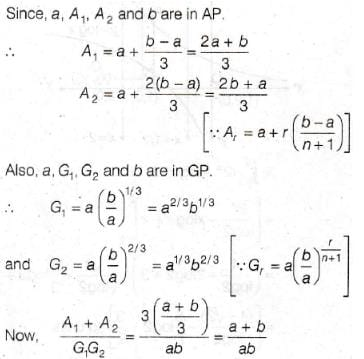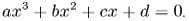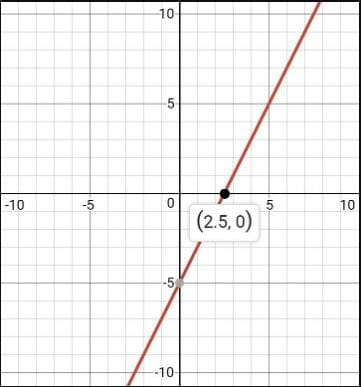Test: Geometric meaning of the Zeroes

# Test: Geometric meaning of the Zeroes - Class 10

Test Description

## 10 Questions MCQ Test Mathematics (Maths) Class 10 - Test: Geometric meaning of the Zeroes

Test: Geometric meaning of the Zeroes for Class 10 2023 is part of Mathematics (Maths) Class 10 preparation. The Test: Geometric meaning of the Zeroes questions and answers have been prepared according to the Class 10 exam syllabus.The Test: Geometric meaning of the Zeroes MCQs are made for Class 10 2023 Exam. Find important definitions, questions, notes, meanings, examples, exercises, MCQs and online tests for Test: Geometric meaning of the Zeroes below.
Solutions of Test: Geometric meaning of the Zeroes questions in English are available as part of our Mathematics (Maths) Class 10 for Class 10 & Test: Geometric meaning of the Zeroes solutions in Hindi for Mathematics (Maths) Class 10 course. Download more important topics, notes, lectures and mock test series for Class 10 Exam by signing up for free. Attempt Test: Geometric meaning of the Zeroes | 10 questions in 10 minutes | Mock test for Class 10 preparation | Free important questions MCQ to study Mathematics (Maths) Class 10 for Class 10 Exam | Download free PDF with solutions
 1 Crore+ students have signed up on EduRev. Have you?
Test: Geometric meaning of the Zeroes - Question 1

### Let A1, A2, be two AM’s and G1, G2 be two GM’s between a and b,then (A1 + A2) / G1G2 is equal to :

Detailed Solution for Test: Geometric meaning of the Zeroes - Question 1Test: Geometric meaning of the Zeroes - Question 2

### If the point (5,0), (0-2) and (3,6) lie on the graph of a polynomial. Then which of the following is a zero of the polynomial?

Detailed Solution for Test: Geometric meaning of the Zeroes - Question 2

We obtain zeros of the polynomial when y=0. Observing the points we see that we have point (5,0) which means when y=0 , x= 5 this means x=5 is a solution of the polynomial.

Test: Geometric meaning of the Zeroes - Question 3

### A polynomial of degree three is called ……

Detailed Solution for Test: Geometric meaning of the Zeroes - Question 3

cubic polynomial is a polynomial of the form. A cubic polynomial is a polynomial of degree 3. A univariate cubic polynomial has the form . An equation involving a cubic polynomial is called a cubic equation. A closed-form solution known as the cubic formula exists for the solutions of an arbitrary cubic equation.

Test: Geometric meaning of the Zeroes - Question 4

A fourth degree polynomial is called

Detailed Solution for Test: Geometric meaning of the Zeroes - Question 4

A polynomial is a mathematical expression which is sum of several terms consisting the same variable in different powers. The degree of the polynomial is the highest power the variable is having in the expression. A quartic polynomial is a polynomial with degree 4. A polynomial with degree 4 can have 0, 1, 2, 3, or 4 roots. There are few methods developed to find the roots of a quartic equation, like Ferrari's solution, changing the equation to depressed form and then solve it or solving a quartic using radicals. These methods are little complex. A quartic function can have 0, 1 or 2 inflection points and have these inflection points implies the concept of golden ratio.

Test: Geometric meaning of the Zeroes - Question 5

The graph of a quadratic polynomial is a parabola which cuts the X- axis at

Test: Geometric meaning of the Zeroes - Question 6

The graph of the polynomial p(x) intersects the x-axis three times in distinct points, then which of the following could be an expression for p(x):

Detailed Solution for Test: Geometric meaning of the Zeroes - Question 6

The polynomial intersects the x-axis three times. A point on x-axis is of the form (a,0) where a is any number.So this means that any point on x axis has y coordinate equal to zero and we know that y=0 is when we have roots of the equation.So since there are three points at which y=0 so we have 3 roots of the equation. No. of roots is equal to the degree of the polynomial. So the degree of the polynomial is 3.

Test: Geometric meaning of the Zeroes - Question 7

The graph of the polynomial f(x) = 2x – 5 crosses the X-axis at the point

Detailed Solution for Test: Geometric meaning of the Zeroes - Question 7

The graph of the polynomial
f (x) = 2x - 5

Method.

Let us consider, y = f (x) = 2x - 5

Now, when x = 0, y = - 5

and when y = 0, x = 5/2

So, we get two points (0, - 5) and (5/2, 0).

We plot the two points on a graph paper considering two rectangular axes XOX' and YOY'. Then, we find a straight line as shown in the graph.

We see that the line intersects x-axis at the point (5/2, 0).Test: Geometric meaning of the Zeroes - Question 8

If “1” is a zero of the polynomial P(a) = x2a2 – 2xa + 3x – 2, then x = ______

Detailed Solution for Test: Geometric meaning of the Zeroes - Question 8

The correct solution of this question is given below:

Here, P(a) = x2a2 - 2xa + 3x - 2

1 is a zero of P(a), so P(1) = 0

Therefore, x212 - 2x.1 + 3x - 2 = 0

x2 + x - 2 = 0

(x + 2)(x - 1) = 0

x = -2, 1

Test: Geometric meaning of the Zeroes - Question 9

Number of observations are 30 and value of arithmetic mean is 15 then sum of all values is

Test: Geometric meaning of the Zeroes - Question 10

If an+1 + bn+1/an + bis geometric mean between a and b, then n =

## Mathematics (Maths) Class 10

115 videos|479 docs|129 tests
Information about Test: Geometric meaning of the Zeroes Page
In this test you can find the Exam questions for Test: Geometric meaning of the Zeroes solved & explained in the simplest way possible. Besides giving Questions and answers for Test: Geometric meaning of the Zeroes, EduRev gives you an ample number of Online tests for practice

## Mathematics (Maths) Class 10

115 videos|479 docs|129 tests